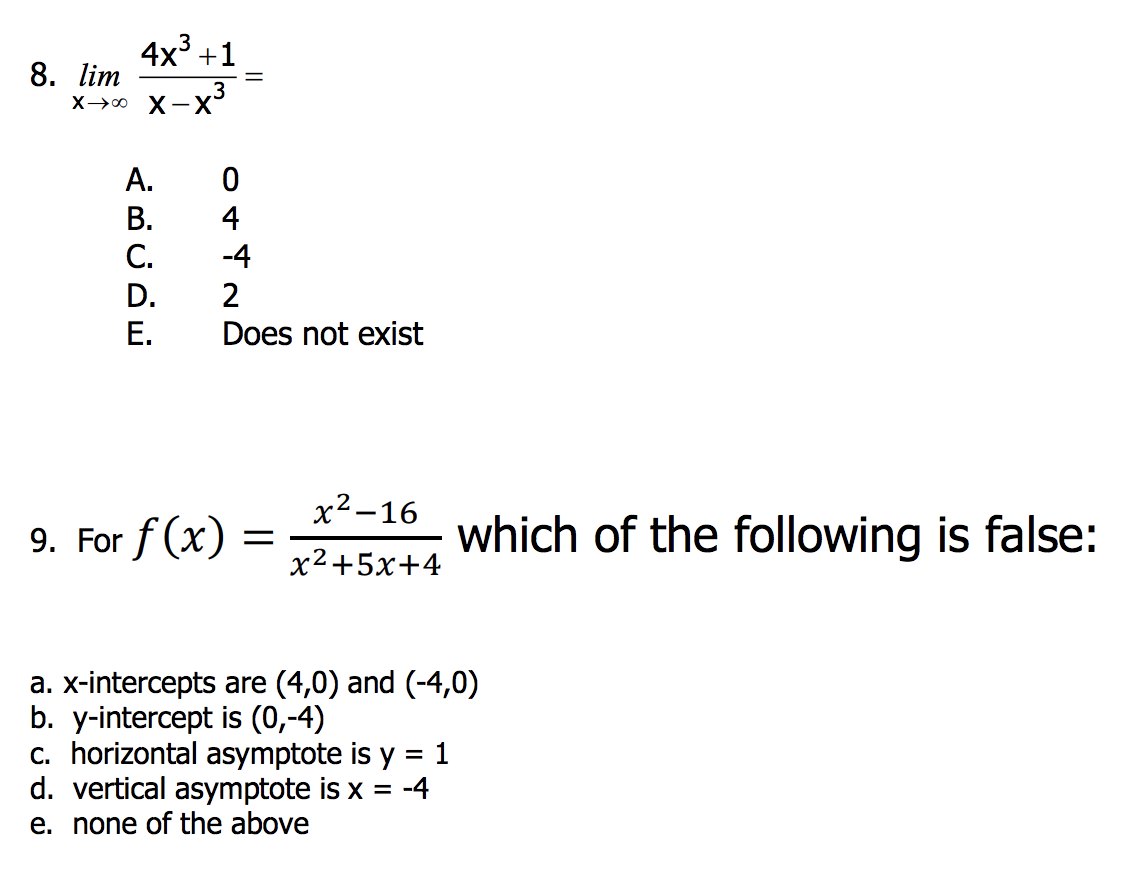# 4x3 +1 8. lim A.0 B.4 C.4 D. 2 E. Does not exist For f 16 which of the following is false: x2+5x+4 a. x-intercepts are (4,0) and (-4,0) b. y-intercept is (0,-4) C. horizontal asymptote is y = 1 d. vertical asymptote is x =-4 e. none of the above

Questionhelp_outlineImage Transcriptionclose4x3 +1 8. lim A.0 B.4 C.4 D. 2 E. Does not exist For f 16 which of the following is false: x2+5x+4 a. x-intercepts are (4,0) and (-4,0) b. y-intercept is (0,-4) C. horizontal asymptote is y = 1 d. vertical asymptote is x =-4 e. none of the above fullscreen